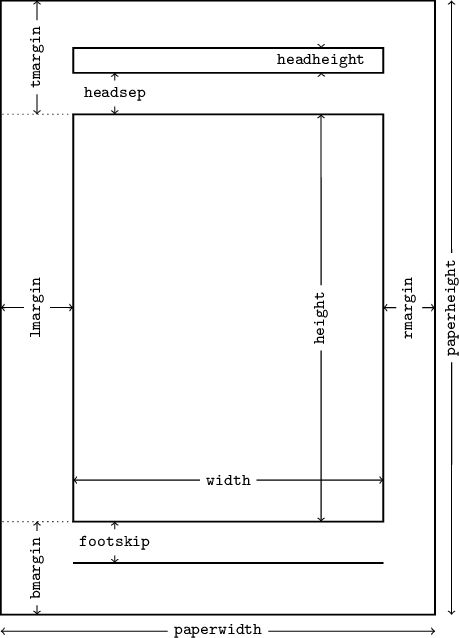# 2015-04-04 The geometry package cheat sheet

One of the problems I’ve always had with the geometry package is that I couldn’t remember the names of many parameters; also, I’ve always forgotten whether the header is included in the height or not by default etc.

Since I (or rather: we, with a coauthor) am/are working on a (yet another) book on LaTeX, I decided that it’s a good opportunity to solve this problem once and for all. So, here’s a diagram showing all the basic geometry parameters.It might be interesting to see the source code for this diagram. It is not extremely elegant (the naming of some of the nodes is far from optimal, though I thought that if I can’t make up reasonable names, I can as well go with numbers). So, here it is. As you can see, it is parametrized, so you can easily change some dimensions (of course, some hand-tuning might be needed). The uppercase doesn’t look great, but it is necessary so that the names do not interfere with (La)TeX dimen registers!

\documentclass{article}

\usepackage{tikz}
\usepackage{lipsum}
\usetikzlibrary{math}
\usetikzlibrary{calc}

\begin{document}

\begin{tikzpicture}[scale=0.035]
\footnotesize
\tikzmath{
\PAPERWIDTH=210;
\PAPERHEIGHT=297;
\LMARGIN=35;
\RMARGIN=25;
\TMARGIN=55;
\BMARGIN=45;
\FOOTSKIP=20;
\WIDTH=\PAPERWIDTH-\LMARGIN-\RMARGIN;
\HEIGHT=\PAPERHEIGHT-\TMARGIN-\BMARGIN;
}

\coordinate(SW) at (0,0);
\coordinate(NE) at (\PAPERWIDTH,\PAPERHEIGHT);
\coordinate(NW) at (SW |- NE);
\coordinate(SE) at (SW -| NE);

\coordinate(sw) at (\LMARGIN,\BMARGIN);
\coordinate(ne) at ($(\LMARGIN,\BMARGIN)+(\WIDTH,\HEIGHT)$);
\coordinate(nw) at (sw |- ne);
\coordinate(se) at (sw -| ne);

\coordinate(fsw) at ($(sw)-(0,\FOOTSKIP)$);
\coordinate(fse) at ($(fsw)+(\WIDTH,0)$);

\coordinate(hsw) at ($(nw)+(0,\HEADSEP)$);
\coordinate(hne) at ($(hsw)+(\WIDTH,\HEADHEIGHT)$);
\coordinate(hnw) at (hsw |- hne);
\coordinate(hse) at (hsw -| hne);

\begin{scope}[thick]
\draw (SW) rectangle (NE);
\draw (sw) rectangle (ne);
\draw (fsw) -- (fse);
\draw (hsw) rectangle (hne);
\end{scope}

\newcommand\hmeasure{% #1: offset, #2: name, #3,#4: coordinates
\node(#2) at ($(#3)!0.5!(#4)+(0,-#1)$) {\texttt{#2}};
\draw[<-] ($(#3)+(0,-#1)$) -- (#2);
\draw[->] (#2) -- ($(#4)+(0,-#1)$);
}
\newcommand\vmeasure{% #1: offset, #2: name, #3,#4: coordinates
\node[rotate=90](#2) at ($(#3)!0.5!(#4)+(#1,0)$) {\texttt{#2}};
\draw[<-] ($(#3)+(#1,0)$) -- (#2);
\draw[->] (#2) -- ($(#4)+(#1,0)$);
}

\hmeasure{paperwidth}{SW}{SE}
\vmeasure{paperheight}{SE}{NE}

\coordinate[yshift=20](sw1) at (sw);
\coordinate[yshift=20](se1) at (se);
\hmeasure{width}{sw1}{se1}

\coordinate[xshift=-30](se2) at (se);
\coordinate[xshift=-30](ne2) at (ne);
\vmeasure{height}{se2}{ne2}

\coordinate[xshift=20](sw3) at (sw);
\coordinate[xshift=20](fsw3) at (fsw);
\hmeasure{footskip}{sw3}{fsw3}

\coordinate[xshift=20](nw4) at (nw);
\coordinate[xshift=20](hsw4) at (hsw);

\coordinate[xshift=-30](hse5) at (hse);
\coordinate[xshift=-30](hne5) at (hne);

\coordinate(W) at ($(SW)!0.5!(NW)$);
\coordinate(w) at ($(sw|-SW)!0.5!(nw|-NW)$);
\vmeasure{lmargin}{W}{w}

\coordinate(E) at ($(SE)!0.5!(NE)$);
\coordinate(e) at ($(se|-SE)!0.5!(ne|-NE)$);
\vmeasure{rmargin}{E}{e}

\coordinate(N) at ($(NW)!0.5!(NW-|nw)$);
\coordinate(n) at ($(nw-|N)$);
\vmeasure{tmargin}{N}{n}
\draw[dotted] (nw) -- (NW|-nw);

\coordinate(S) at ($(SW)!0.5!(SW-|sw)$);
\coordinate(s) at ($(sw-|S)$);
\vmeasure{bmargin}{S}{s}
\draw[dotted] (sw) -- (SW|-sw);
\end{tikzpicture}

\end{document}